# Synchrotron radiation

Synchrotron radiation
This article concerns the physical phenomenon of synchrotron radiation. For details on the production of this radiation and applications in laboratories, see Synchrotron light source.

The electromagnetic radiation emitted when charged particles are accelerated radially ($\mathbf{a}\perp \mathbf{v}$) is called synchrotron radiation. It is produced in synchrotrons using bending magnets, undulators and/or wigglers. It is similar to cyclotron radiation except that is generated by the acceleration of ultrarelativistic (moving near the speed of light) charged particles through magnetic fields. This may be achieved artificially in synchrotron or storage rings, or naturally by fast electrons moving through magnetic fields in space. The radiation produced in this way has a characteristic polarization and can range over the entire electromagnetic spectrum.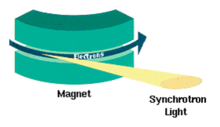Synchrotron radiation from a bending magnetSynchrotron radiation from an undulator

## HistoryGeneral Electric synchrotron accelerator built in 1946, the origin of the discovery of synchrotron radiation. The arrow indicates the evidence of radiation.

Synchrotron radiation was named after its discovery in a General Electric synchrotron accelerator built in 1946 and announced in May 1947 by Frank Elder, Anatole Gurewitsch, Robert Langmuir, and Herb Pollock in a letter entitled "Radiation from Electrons in a Synchrotron". Pollock recounts:

"On April 24, Langmuir and I were running the machine and as usual were trying to push the electron gun and its associated pulse transformer to the limit. Some intermittent sparking had occurred and we asked the technician to observe with a mirror around the protective concrete wall. He immediately signaled to turn off the synchrotron as "he saw an arc in the tube." The vacuum was still excellent, so Langmuir and I came to the end of the wall and observed. At first we thought it might be due to Cherenkov radiation, but it soon became clearer that we were seeing Ivanenko and Pomeranchuk radiation."

## Properties of synchrotron radiation

1. Broad Spectrum (which covers from microwaves to hard X-rays): the users can select the wavelength required for their experiment.
2. High Flux: high intensity photon beam allows rapid experiments or use of weakly scattering crystals.
3. High Brilliance: highly collimated photon beam generated by a small divergence and small size source (spatial coherence)
4. High Stability: submicron source stability
5. Polarization: both linear and circular
6. Pulsed Time Structure: pulsed length down to tens of picoseconds allows the resolution of process on the same time scale.

## Emission mechanism

When high-energy particles are in rapid motion, including electrons forced to travel in a curved path by a magnetic field, synchrotron radiation is produced. This is similar to a radio antenna, but with the difference that, in theory, the relativistic speed will change the observed frequency due to the Doppler effect by the Lorentz factor, γ. Relativistic time contraction then bumps the frequency observed in the lab by another factor of γ, thus multiplying the GHz frequency of the resonant cavity that accelerates the electrons into the X-ray range. The radiated power is given by the relativistic Larmor formula while the force on the emitting electron is given by the Abraham-Lorentz-Dirac force. The radiation pattern can be distorted from an isotropic dipole pattern into an extremely forward-pointing cone of radiation. Synchrotron radiation is the brightest artificial source of X-rays. The planar acceleration geometry appears to make the radiation linearly polarized when observed in the orbital plane, and circularly polarized when observed at a small angle to that plane. Amplitude and frequency are however focussed to the polar ecliptic.

## Formulation

### Liénard–Wiechert Field$\mathbf{B}(\mathbf{r},t)=-\frac{\mu_0q}{4\pi}\left[\frac{c\,\hat{\mathbf{n}}\times\vec{\beta}}{\gamma^2R^2(1-\vec{\beta}\mathbf{\cdot}\hat{\mathbf{n}})^3}+\frac{\hat{\mathbf{n}}\times[\,\dot{\vec{\beta}}+\hat{\mathbf{n}}\times(\vec{\beta}\times\dot{\vec{\beta}})]}{R\,(1-\vec{\beta}\mathbf{\cdot}\hat{\mathbf{n}})^3}\right]_{\mathrm{retarded}} \qquad (1)$$\mathbf{E}(\mathbf{r},t)=\frac{q}{4\pi\varepsilon_0}\left[\frac{\hat{\mathbf{n}}-\vec{\beta}}{\gamma^2R^2(1-\vec{\beta}\mathbf{\cdot}\hat{\mathbf{n}})^3}+\frac{\hat{\mathbf{n}}\times[(\hat{\mathbf{n}}-\vec{\beta})\times\dot{\vec{\beta}}\,]}{c\,R\,(1-\vec{\beta}\mathbf{\cdot}\hat{\mathbf{n}})^3}\right]_{\mathrm{retarded}} \qquad \qquad (2)$

where$\mathbf R(t')=\mathbf r-\mathbf r_0(t'),$$R(t')=|\mathbf R|,$$\hat{\mathbf n}(t')=\mathbf R/R,$

which is the unit vector between the observation point and the position of the charge at the retarded time, and t' is the retarded time.

In equation (1), and (2), the first terms fall off as the inverse square of the distance from the particle, and this first term is called the generalized Coulomb field or velocity field. And the second terms fall off as the inverse first power of the distance from the source, and it is called the radiation field or acceleration field. If we ignore the velocity field, the radial component of Poynting's Vector resulted from the Liénard–Wiechert field can be calculated to be$[\mathbf{S\cdot}\hat{\mathbf{n}}]_{\mathrm{retarded}} = \frac{q^2}{16\pi^2\varepsilon_0 c}\left\{\frac{1}{R^2}\left|\frac{\hat{\mathbf{n}}\times[(\hat{\mathbf{n}}-\vec{\beta})\times\dot{\vec{\beta}}]}{(1-\vec{\beta}\mathbf{\cdot}\hat{\mathbf{n}})^3}\right|^2\right\}_{\mathrm{not retarded}} \qquad \qquad (3)$

Note that

• The spatial relationship between$\vec{\beta}$ and$\dot{\vec{\beta}}$ determines the detailed angular power distribution.
• The relativistic effect of transforming from the rest frame of the particle to the observer's frame manifests itself by the presence of the factors$(1-\vec{\beta}\mathbf{\cdot}\vec{\mathbf{n}})$ in the denominator of Eq. (3).
• For ultrarelativistic particles the latter effect dominates the whole angular distribution.

The energy radiated into per solid angle during a finite period of acceleration from t' = T1 to t' = T2 is$\frac{\mathrm{d}P}{\mathrm{d}\mathit{\Omega}} = R(t')^2\,[\mathbf{S}(t')\mathbf{\cdot}\hat{\mathbf{n}}(t')]\,\frac{\mathrm{d}t}{\mathrm{d}t'} = R(t')^2\,\mathbf{S}(t')\mathbf{\cdot}\hat{\mathbf{n}}(t')\,[1-\vec{\beta}(t')\mathbf{\cdot}\hat{\mathbf{n}}(t')]$$= \frac{q^2}{16\pi^2\varepsilon_0c}\,\frac{|\hat{\mathbf{n}}(t')\times\{[\hat{\mathbf{n}}(t')-\vec{\beta}(t')]\times\dot{\vec{\beta}}(t')\}|^2}{[1-\vec{\beta}(t')\mathbf{\cdot}\vec{\mathbf{n}}(t')]^5} \qquad \qquad (4)$

Integrating Eq. (4) over the all solid angles, we got relativistic generalization of Larmor's formula$P=\frac{e^2}{6\pi \varepsilon _0 c}\gamma ^6 \left [ \left | \dot{\vec{\beta }} \right |^2 -\left | \vec{\beta}\times \dot{\vec{\beta }}\right | \right ]\qquad (5)$

However, this also can be derived by relativistic transformation of the 4-acceleration in Larmor's formula.

### Velocity$\perp$acceleration: synchrotron radiation

When the charge is in instantaneous circular motion with its acceleration$\dot{\vec{\beta}}$ perpendicular to its velocity$\vec{\beta}$. Choosing a coordinate system such that instantaneously$\vec{\beta}$ is in the z direction and$\dot{\vec{\beta}}$ is in the x direction. With the customary polar angles$\theta\,\mathrm{,}\,\phi$ defining the direction of observation, the general formula Eq. (4) reduces to$\frac{\mathrm{d}P}{\mathrm{d}\mathit{\Omega}} = \frac{q^2}{16\pi^2\epsilon_0 c}\frac{|\dot{\vec{\beta }}|^2}{(1-\beta\cos\theta)^3}\left[1-\frac{\sin^2\theta\cos^2\phi}{\gamma^2(1-\beta\cos\theta)^2}\right] \qquad (6)$

In the relativistic limit (γ > > 1), the angular distribution can be written approximately$\frac{\mathrm{d}P}{\mathrm{d}\mathit{\Omega}} \simeq \frac{2}{\pi}\frac{e^2}{c^3}\gamma^6\frac{|\dot{\mathbf v}|^2}{(1+\gamma^2\theta^2)^3}\left[1-\frac{4\gamma^2\theta^2\cos^2\phi}{(1+\gamma^2\theta^2)^2}\right] \qquad \qquad (7)$

The factors (1 − βcos θ) in the denominators tip the angular distribution forward into a narrow cone like the beam of a headlight pointing ahead of the particle. The figure of the angular distribution (dP/dΩ vs. γθ plot) has a sharp peak around θ = 0.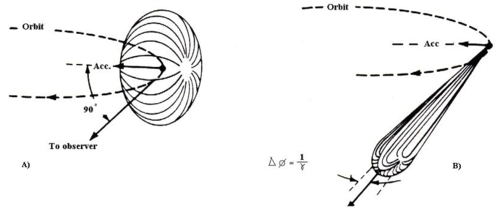When the electron velocity approaches the speed of light, the emission pattern is sharply collimated forward.

Integrating over the whole solid angle we obtain the total power radiated by one electron$P=\frac{e^2}{6\pi\epsilon_0c}\left | \dot{\vec{\beta }} \right |^2\gamma ^4=\frac{e^2c}{6\pi\epsilon_0}\frac{\gamma ^4}{\rho ^2}=\frac{e^4}{6\pi\epsilon_0m^4c^5}E^2B^2\qquad (8)$

Note that radiated power is proportional to 1 / m4, 1 / ρ2, and B2. Because of synchrotron radiation's high power, the surface of the vacuum chamber hit by synchrotron radiation must be cooled.

### Radiation integral

The energy received by an observer (per unit solid angle at the source) is$\frac{d^2W}{d\Omega }=\int_{-\infty }^{\infty }\frac{d^2P}{d\Omega }dt=c\varepsilon _0\int_{-\infty }^{\infty }\left | R\vec{E}(t) \right |^2dt$

Using the Fourier Transformation we move to the frequency space$\frac{d^2W}{d\Omega }=2c\varepsilon _0\int_{0 }^{\infty }\left | R\vec{E}(w) \right |^2dw$

Angular and frequency distribution of the energy received by an observer (consider only the radiation field)$\frac{d^3W}{d\Omega dw }=2c\varepsilon _0R^2\left | \vec{E}(w) \right |^2=\frac{e^2}{4\pi\varepsilon_0 4\pi^2 c}\left | \int_{-\infty}^{\infty}\frac{\hat{n}\times\left [ \left ( \hat{n}-\vec{\beta } \right )\times\dot{\vec{\beta }} \right ]}{\left ( 1-\hat{n}\cdot \vec{\beta } \right )^2}e^{iw(t-\hat{n}\cdot\vec{r}(t)/c)}dt\right |^2 \qquad (9)$

Therefore, if we know the particle's motion, cross products term, and phase factor, we could calculate the radiation integral. However, calculations are generally quite lengthy (even for simple cases as for the radiation emitted by an electron in a bending magnet, they require Airy function or the modified Bessel functions).

### Example 1: bending magnet

#### Radiation integral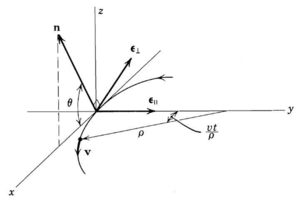Trajectory of the arc of circumference

Trajectory of the arc of circumference is$\vec{r}(t)=\left ( \rho\left ( 1-cos\frac{\beta c}{\rho}t \right ), sin\frac{\beta c}{\rho}t, 0 \right)$

In the limit of small angles we compute$\hat{n}\times\left ( \hat{n}\times\vec{\beta} \right ) =\beta\left [ -\vec{\varepsilon }_\parallel \sin\left ( \frac{\beta c t}{\rho} \right )+\vec{\varepsilon}_\perp \cos\left ( \frac{\beta c t}{\rho}\right )\sin\theta \right ]$$w\left ( t-\frac{\hat{n}\cdot \vec{r}(t)}{c} \right ) = w\left [ t-\frac{\rho}{c}\sin\left ( \frac{\beta c t}{\rho} \right )\cos\theta \right ]$

Substituting into the radiation integral and introducing$\xi =\frac{\rho w}{3c\gamma^3}\left ( 1+\gamma^2 \theta^2 \right )^{3/2}$$\frac{d^3 W}{d\Omega dw}=\frac{e^2}{16\pi^3\varepsilon_0 c} \left ( \frac{2w\rho}{3c\gamma^2} \right )^2 \left ( 1+\gamma^2 \theta^2 \right )^2 \left [ K_{2/3}^2(\xi ) + \frac{\gamma^2 \theta^2}{1+\gamma^2 \theta^2}K_{1/3}^2(\xi)\right ]\qquad (10)$

,where the function K is modified Bessel function.

#### Frequency distribution of radiated energy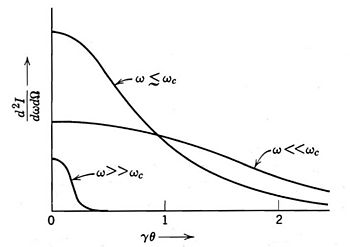Angular distribution of radiated energy

From the Eq.(10), we observe that the radiation intensity is negligible for$\xi\gg 1$. Critical frequency is defined as the frequency when$\xi=\frac{1}{2}$ and θ = 0. So,$w_c=\frac{3}{2}\frac{c}{\rho}\gamma^3$

,and critical angle is defined as$\theta_c=\frac{1}{\gamma}\left ( \frac{w_c}{w} \right )^{1/3}$

For frequencies much larger than the critical frequency and angles much larger than the critical angle, the synchrotron radiation emission is negligible.

Integrating on all angles, we get the frequency distribution of the energy radiated.$\frac{dW}{dw}=\oint \frac{d^3 W}{dw d\Omega }d\Omega =\frac{\sqrt{3}e^2}{4\pi\varepsilon_0 c}\gamma\frac{w}{w_c}\int_{w/w_c}^{\infty}K_{5/3}(x)dx$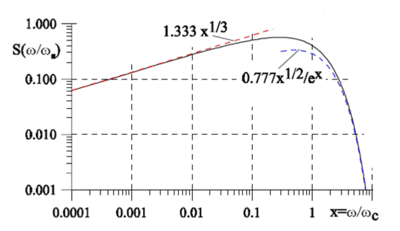Frequency distribution of radiated energy

If we define$S(y)\equiv \frac{9\sqrt{3}}{8\pi}y\int_{y}^{\infty}K_{5/3}(x)dx$$\int_{0}^{\infty}S(y)dy=1$

,where$y=\frac{w}{w_c}$. Then,$\frac{dW}{dw}=\frac{2e^2 \gamma}{9 \varepsilon_0c}S(y)\qquad (11)$

Note that$\frac{dW}{dw}\approx \frac{e^2}{4\pi \varepsilon_0c}\left ( \frac{w \rho}{c} \right )^{1/3}$, if$w\gg w_c$, and$\frac{dW}{dw}\approx \sqrt{\frac{3\pi}{2}}\frac{e^2}{4\pi\varepsilon_0 c}\gamma\left ( \frac{w}{w_c} \right )^2 e^{-w/w_c}$, if$w\ll w_c$

Formula for spectral distribution of synchrotron radiation, given above, can be expressed in terms of rapidly coverged integral with no special functions involved  (see also modified Bessel functions ) by means of the relation:$\int_{\xi}^\infty K_{5/3} (x) dx = \frac{1}{ \sqrt{3}} \, \int_0^\infty \, \frac{9+36x^2+16x^4}{(3+4x^2) \sqrt{1+x^2/3}} \exp \left[- \xi \left(1+\frac{4x^2}{3}\right) \sqrt{1+\frac{x^2}{3}} \right] \ dx$

#### Synchrotron radiation emission as a function of the beam energy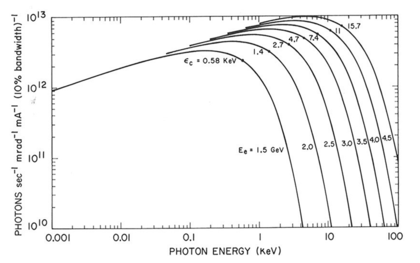Relationship between power radiated and the photon energy

First, define the critical photon energy as$\varepsilon_c=\hbar w_c=\frac{3}{2}\frac{\hbar c}{\rho}\gamma^3$

Then, the relationship between radiated power and photon energy is shown in the graph on the rightside. The higher the critical energy, the more photons wih high energies are generated. Note that, there is no dependence on the energy at longer wavelength.

#### Polarization of synchrotron radiation

In Eq.(10), the first term$K_{2/3}^2(\xi)$ is the radiation power with polarization in the orbit plane, and the second term$\frac{\gamma^2 \theta^2}{1+\gamma^2 \theta^2}K_{1/3}^2(\xi)$ is the polarization orthogonal to the orbit plane. In the orbit plane θ = 0, the polarization is purely horizontal. Integrating on all frequencies, we get the angular distribution of the energy radiated$\frac{d^2 W}{d\Omega }=\int_{0}^{\infty}\frac{d^3W}{dwd\Omega }dw =\frac{7e^2 \gamma^5}{64\pi\varepsilon_0\rho}\frac{1}{(1+\gamma^2\theta^2)^{5/2}}\left [1+\frac{5}{7}\frac{\gamma^2\theta^2}{1+\gamma^2\theta^2} \right ] \qquad (12)$

Integrating on all the angles, we find that seven times as much energy is radiated with parallel polarization as with perpendicular polarization. The radiation from a relativistically moving charge is very strongly, but not completely, polarized in the plane of motin.

### Example 2: undulator

#### Solution of equation of motion and undulator equation

Undulator is consist of periodic array of magnets, so that they provide a sinusoidal magnetic field.$\vec{B}=\left ( 0, B_0 \sin(k_u z),0 \right )$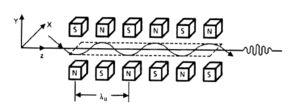undulator

Solution of equation of motion is$\vec{r}(t)=\frac{\lambda_u K}{2\pi\gamma}\sin w_ut\cdot \hat{x} +\left ( \bar{\beta_z}ct+\frac{\lambda_uK^2}{16\pi\gamma^2}\cos(2w_ut) \right )\cdot \hat{z}$

where,$K=\frac{eB_0\lambda_u}{2\pi mc}$ , and$\bar{\beta_z}=1-\frac{1}{2\gamma^2}\left ( 1+\frac{K^2}{2} \right )$

,and the parameter K is called the undulator parameter.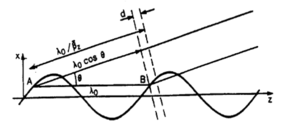Constructive interference of the beam in the undulator

Condition for the constructive interference of radiation emitted at different poles is$d=\frac{\lambda_u}{\bar{\beta}}-\lambda_u\cos\theta=n\lambda$

Therefore,$\lambda_n = \frac{\lambda_u}{2\gamma^2n}\left ( 1+\frac{K^2}{2}+\gamma^2\theta^2 \right )\qquad (13)$

This equation is called the undulator equation.

#### Radiation from the undulator

Radiation integral is$\frac{d^3W}{d\Omega dw }=\frac{e^2}{4\pi\varepsilon_0 4\pi^2 c}\left | \int_{-\infty}^{\infty}\frac{\hat{n}\times\left [ \left ( \hat{n}-\vec{\beta } \right )\times\dot{\vec{\beta }} \right ]}{\left ( 1-\hat{n}\cdot \vec{\beta } \right )^2}e^{iw(t-\hat{n}\cdot\vec{r}(t)/c)}dt\right |^2$

Using the periodicity of the trajectory, we can split the radiation integral into a sum over Nu terms.$\frac{d^3W}{d\Omega dw }=\frac{e^2w^2}{4\pi\varepsilon_0 4\pi^2 c}\left | \int_{-\lambda_u/2\bar{\beta}c}^{\lambda_u/2\bar{\beta}c}\hat{n}\times\left ( \hat{n}\times\vec{\beta} \right )e^{iw(t-\hat{n}\cdot\vec{r}(t)/c)}dt\right|^2 \left|1+e^{i\delta}+e^{2i\delta}+\cdots +e^{i(N_u-1)\delta} \right |^2 \qquad (14)$

, where$\bar{\beta}=\beta\left ( 1-\frac{K^2}{4\gamma^2} \right )$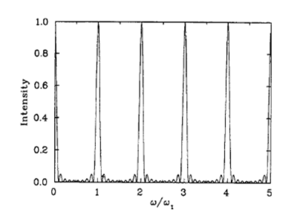Peak frequencies become sharp as the number N increases

, and$\delta=\frac{2\pi w}{w_{res} (\theta)}$,$w_{res}(\theta)=\frac{2\pi c}{\lambda_{res}(\theta)}$,　　and$\lambda_{res}(\theta)=\frac{\lambda_u}{2\gamma^2}\left ( 1+\frac{K^2}{2}+\gamma^2\theta^2 \right )$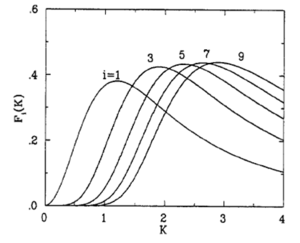Only odd harmonics are radiated on-axis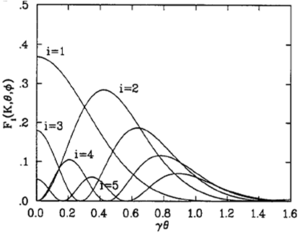Off-axis radiation contains many harmonics

The radiation integral in an undulator can be written as$\frac{d^3 W}{d\Omega dw}=\frac{e^2\gamma^2N^2}{4\pi\varepsilon_0 c} L\left ( N\frac{\Delta w_n}{w_{res}(\theta)} \right ) F_n (K, \theta, \phi) \qquad (15)$

The sum of δ generates a series of sharp peaks in the frequency spectrum harmonics of fundamental wavelength$L\left ( N\frac{\Delta w_k}{w_{res}(\theta)} \right ) =\frac{\sin^2\left ( N\pi\Delta w_k / w_{res}(\theta) \right )}{N^2 \sin^2\left ( \pi\Delta w_k/w_{res}(\theta) \right )}$

,and Fn depends on the angles of observations and K$F_n(K,\theta,\phi)\propto \left | \int_{-\lambda_u/2\bar{\beta} c}^{\lambda_u/2\bar{\beta} c}\hat{n}\times\left ( \hat{n}\times\vec{\beta} \right )e^{iw(t-\hat{n}\cdot\vec{r}(t)/c)}dt\right|^2$

On the axis(θ = 0, φ = 0), the radiation integral becomes$\frac{d^3 W}{d\Omega dw}=\frac{e^2\gamma^2N^2}{4\pi\varepsilon_0 c}L\left ( N\frac{\Delta w_n}{w_{res}(0)} \right )F_n(K,0,0)$

and,$F_n(K,0,0)=\frac{n^2K^2}{1+K^2/2} \left[J_{\frac{n+1}{2}}(Z)-J_{\frac{n-1}{2}}(Z) \right ]^2$

, where$Z=\frac{nK^2}{4(1+K^2/2)}$

Note that only odd harmonics are radiated on-axis, and as K increases higher harmonic becomes stronger.

## Synchrotron radiation from accelerators

Synchrotron radiation may occur in accelerators either as a nuisance, causing undesired energy loss in particle physics contexts, or as a deliberately produced radiation source for numerous laboratory applications. Electrons are accelerated to high speeds in several stages to achieve a final energy that is typically in the GeV range. In the LHC proton bunches also produce the radiation at increasing amplitude and frequency as they accelerate with respect to the vacuum field, propagating photoelectrons, which in turn propagate secondary electrons from the pipe walls with increasing frequency and density up to 7x1010. Each proton may lose 6.7keV per turn due to this phenomenon.

## Synchrotron radiation in astronomy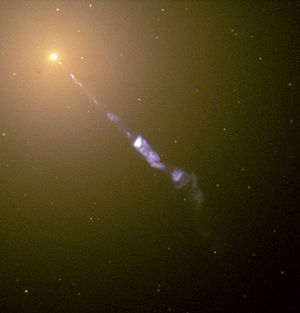M87's Energetic Jet, HST image. The blue light from the jet emerging from the bright AGN core, towards the lower right, is due to synchrotron radiation.

Synchrotron radiation is also generated by astronomical objects, typically where relativistic electrons spiral (and hence change velocity) through magnetic fields. Two of its characteristics include non-thermal power-law spectra, and polarization.

### History

It was first detected in a jet emitted by M87 in 1956 by Geoffrey R. Burbidge, who saw it as confirmation of a prediction by Iosif S. Shklovsky in 1953, but it had been predicted several years earlier by Hannes Alfvén and Nicolai Herlofson  in 1950.

T. K. Breus noted that questions of priority on the history of astrophysical synchrotron radiation is quite complicated, writing:

"In particular, the Russian physicist V.L. Ginzburg broke his relationships with I.S. Shklovsky and did not speak with him for 18 years. In the West, Thomas Gold and Sir Fred Hoyle were in dispute with H. Alfven and N. Herlofson, while K.O. Kiepenheuer and G. Hutchinson were ignored by them."

Supermassive black holes have been suggested for producing synchrotron radiation, by ejection of jets produced by gravitationally accelerating ions through the super contorted 'tubular' polar areas of magnetic fields. Such jets, the nearest being in Messier 87, have been confirmed by the Hubble telescope as apparently superluminal, travelling at 6×c (six times the speed of light) from our planetary frame. A number of inconsistent solutions have been offered but it is suggested by both the DFM (Discrete Field Model) and 'Einstein Aether theory' to be the columnar effect of charged particles following and travelling within previous 'tubes' of particles, all travelling at 'c' locally with respect to the previous particles. The observations are of change of position, with light from the observations arriving at 'c', so the second postulate of SR (invariance of speed of light) would not be violated.

### Pulsar wind nebulaeThe bluish glow from the central region of the nebula is due to synchrotron radiation.

A class of astronomical sources where synchrotron emission is important is the pulsar wind nebulae, or plerions, of which the Crab nebula and its associated pulsar are archetypal. Pulsed emission gamma-ray radiation from the Crab has recently been observed up to ≥25 GeV, probably due to synchrotron emission by electrons trapped in the strong magnetic field around the pulsar. Polarization in the Crab at energies from 0.1 to 1.0 MeV illustrates a typical synchrotron radiation.

## See also

Wikimedia Foundation. 2010.

### Look at other dictionaries:

• synchrotron radiation — n. the electromagnetic radiation given off by high energy particles, as electrons, as they spiral at a speed close to the speed of light in a strong magnetic field, as in a synchrotron or in a galaxy …   English World dictionary

• synchrotron radiation — electromagnetic radiation emitted by charged particles as they pass through magnetic fields. [1960 65] * * * Electromagnetic radiation emitted by charged particles that are moving at speeds close to that of light when their paths are altered. It… …   Universalium

• synchrotron radiation — sinchrotroninis spinduliavimas statusas T sritis fizika atitikmenys: angl. acceleration radiation; synchrotron radiation vok. Synchrotronstrahlung, f; Zyklotronstrahlung, f rus. синхротронное излучение, n pranc. rayonnement synchrotron, m …   Fizikos terminų žodynas

• synchrotron radiation — sinchrotroninis spinduliavimas statusas T sritis chemija apibrėžtis Elektringųjų dalelių, kertančių magnetinį lauką greičiu, beveik lygiu šviesos greičiui, sukeltas elektromagnetinis spinduliavimas. atitikmenys: angl. acceleration radiation;… …   Chemijos terminų aiškinamasis žodynas

• synchrotron radiation — noun the electromagnetic radiation emitted by the accelerating charged particles in a synchrotron that are moving at near the speed of light Syn: synchrotron light …   Wiktionary

• synchrotron radiation — noun Etymology: from its having been first observed in a synchrotron Date: 1956 radiation emitted by high energy charged relativistic particles (as electrons) when they are accelerated by a magnetic field (as in a nebula) …   New Collegiate Dictionary

• synchrotron radiation — /ˌsɪŋkrətrɒn reɪdiˈeɪʃən/ (say .singkruhtron raydee ayshuhn) noun the emission of electromagnetic radiation by interstellar gas clouds in radio galaxies, believed to be analogous to the light emitted by high energy electrons in a synchrotron …   Australian-English dictionary

• synchrotron radiation — noun Etymology: so called from its having been first observed in a synchrotron : electromagnetic radiation emitted by high energy charged relativistic particles (as electrons) when they are accelerated by a magnetic field (as in a nebula) …   Useful english dictionary

• synchrotron radiation — noun Physics polarized radiation emitted by a charged particle spinning in a magnetic field …   English new terms dictionary

• Synchrotron Radiation Source — The Synchrotron Radiation Source (SRS) at the Daresbury Laboratory in Cheshire, England was a second generation [cite web |url=http://www.srs.ac.uk/srs/news extras/news extra5.htm |title = Synchrotron Radiation Source, Daresbury Laboratory World… …   Wikipedia

### Share the article and excerpts

##### Direct link
Do a right-click on the link above
and select “Copy Link”